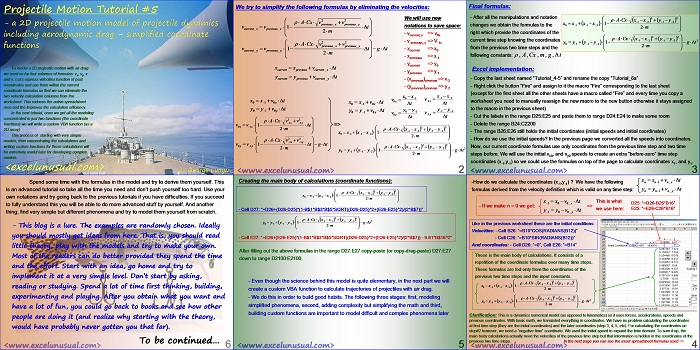# 2D Projectile Motion Tutorial #6

This tutorial simplifies the previous model and manages to describe the (x,y) flight coordinates using just two formulas placed on columns D and E.

A custom VBA trajectory function will be introduced in the next section which preserves the effects of gravity and aerodynamic drag.[sociallocker][/sociallocker]

## Projectile Motion Tutorial #5

by George Lungu

– a 2D projectile motion model of projectile dynamics
including aerodynamic drag – simplified coordinate
functions

To model a 2D projectile motion with air drag
we used so far four columns of formulas: vx, vy, x
and y.

Let’s express velocities function of past
coordinates and use them within the current
coordinate formulas so that we can eliminate the
two velocity calculation columns from the
worksheet.

area and the improves the calculation efficiency.

In the next tutorial, once we got all the modeling
concentrated in just two functions (the coordinate
functions) we will write a custom VBA function (as a
2D array).

This process of starting with very simple
models, then concentrating the calculations and
writing custom functions for those calculations will
be extremely useful later for developing complex
models.

<excelunusual.com>

#### We try to simplify the following formulas by eliminating the velocities:

We will use new formulas as shown:

<www.excelunusual.com> 2

### Final formulas:

-After all the manipulations and notation changes we obtain the formulas to the right which provide the coordinates of the current time step knowing the coordinates from the previous two time steps and the following constants: tgm,CxA, rho,delta

### Excel implementation:

– Copy the last sheet named “Tutorial_4-5” and rename the copy “Tutorial_6a”

– Right click the button “Fire” and assign to it the macro “Fire” corresponding to the last sheet
(except for the first sheet all the other sheets have a macro called “Fire” and every time you copy a
worksheet you need to manually reassign the new macro to the new button otherwise it stays assigned
to the macro in the previous sheet)

– Cut the labels in the range D25:E25 and paste them to range D24:E24 to make some room

– Delete the range B24:C2200

– The range B26:E26 still holds the initial coordinates (initial speeds and initial coordinates)

– How do we use the initial speeds? In the previous page we converted all the speeds into coordinates.

Now, our current coordinate formulas use only coordinates from the previous time step and two time
steps before. We will use the initial vx0, and vy0 speeds to create an extra “before-zero” time step
coordinates (x-1,y-1) so we could use the formulas on top of the page to calculate coordinates x1, and y1.

<www.excelunusual.com> 3

-How do we calculate the coordinates (x-1,y-1) ? We have the following n, n+1 n_x
formulas derived from the velocity definition which is valid on any time step: y , y ,v+t

#### Like in the previous worksheet these are the initial conditions:

And coordinates: – Cell D26: “=0”, Cell E26: “=B14”

These is the main body of calculations. It consists of a repetition of the coordinate formulas over many time steps.

These formulas are fed only from the coordinates of the previous two time steps and the input constants.

Clarification: This is a dynamics numerical model (as opposed to kinematics) so it uses forces, accelerations, speeds and
previous coordinates. With basic math, we translated everything in coordinates. We have no problem calculating the coordinates
at first time step (they are the initial coordinates) and the later coordinates (step 3, 4, 5, etc). For calculating the coordinates on
step#2 however, we need a “negative time” coordinate. We used the initial speed to expand the time domain. To sum it up, the
main body calculations actually need the velocities of the previous time step but that information is hidden in the coordinates of the
previous two time steps. In the next page you can see the exact spreadsheet formulas used =>

<www.excelunusual.com> 4

### Creating the main body of calculations (coordinate functions):

– Cell D27: “=D26+(D26-D25)*(1-B\$1*B\$3*B\$5*SQRT((D26-D25)^2+(E26-E25)^2)/(2*B\$7))”

– Cell E27: “=E26+(E26-E25)*(1-B\$1*B\$3*B\$5*SQRT((D26-D25)^2+(E26-E25)^2)/(2*B\$7)) – 9.81*B\$16^2”

After filling out the above formulas in the range D27:E27 copy-paste (or copy-drag-paste) D27:E27
down to range D2100:E2100.

– Even though the science behind this model is quite elementary, in the next part we will
create a custom VBA function to calculate trajectories of projectiles with air drag.

– We do this in order to build good habits. The following three stages: first, modeling
simplified phenomena, second, adding complexity but simplifying the math and third,
building custom functions are important to model difficult and complex phenomena later

<www.excelunusual.com> 5

Spend some time with the formulas in the model and try to derive them yourself.

This is an advanced tutorial so take all the time you need and don’t push yourself too hard.

Use your own notations and try going back to the previous tutorials if you have difficulties. If you succeed
to fully understand this you will be able to do more advanced stuff by yourself. And another
thing, find very simple but different phenomena and try to model them yourself from scratch.

– This blog is a lure. The examples are randomly chosen. Ideally
you should mostly get ideas from here. That is, you should read
little theory, play with the models and try to make your own.

Most of the readers can do better provided they spend the time
and the effort. Start with an idea, go home and try to
implement it at a very simple level.

Don’t start by asking, reading or studying. Spend a lot of time first thinking, building,
experimenting and playing.

After you obtain what you want and have a lot of fun, you could go back to books and see how other
people are doing it (and realize why starting with the theory, would have probably never gotten you that far).

To be continued…

George Lungu <www.excelunusual.com>